Question

# You are given a 0.553 gram sample of an unknown metal carbonate. You add some of...

You are given a 0.553 gram sample of an unknown metal carbonate. You add some of the sample to a 10.0 mL sample of 0.255 M HNO3. When you have added exactly enough sample to react with all the acid, you reweigh your remaining sample and find its mass is 0.364 grams.

(a). How many moles of acid reacted with the sample

(b). How many grams of sample reacted with the acid?

(c). How many moles of the unknown carbonate reacted with the acid?

(d). What is the molar mass of the sample?

(e). What is the identity of the unknown carbonate? The carbonate is a group 1 or group 2 carbonate. You need to enter a formula.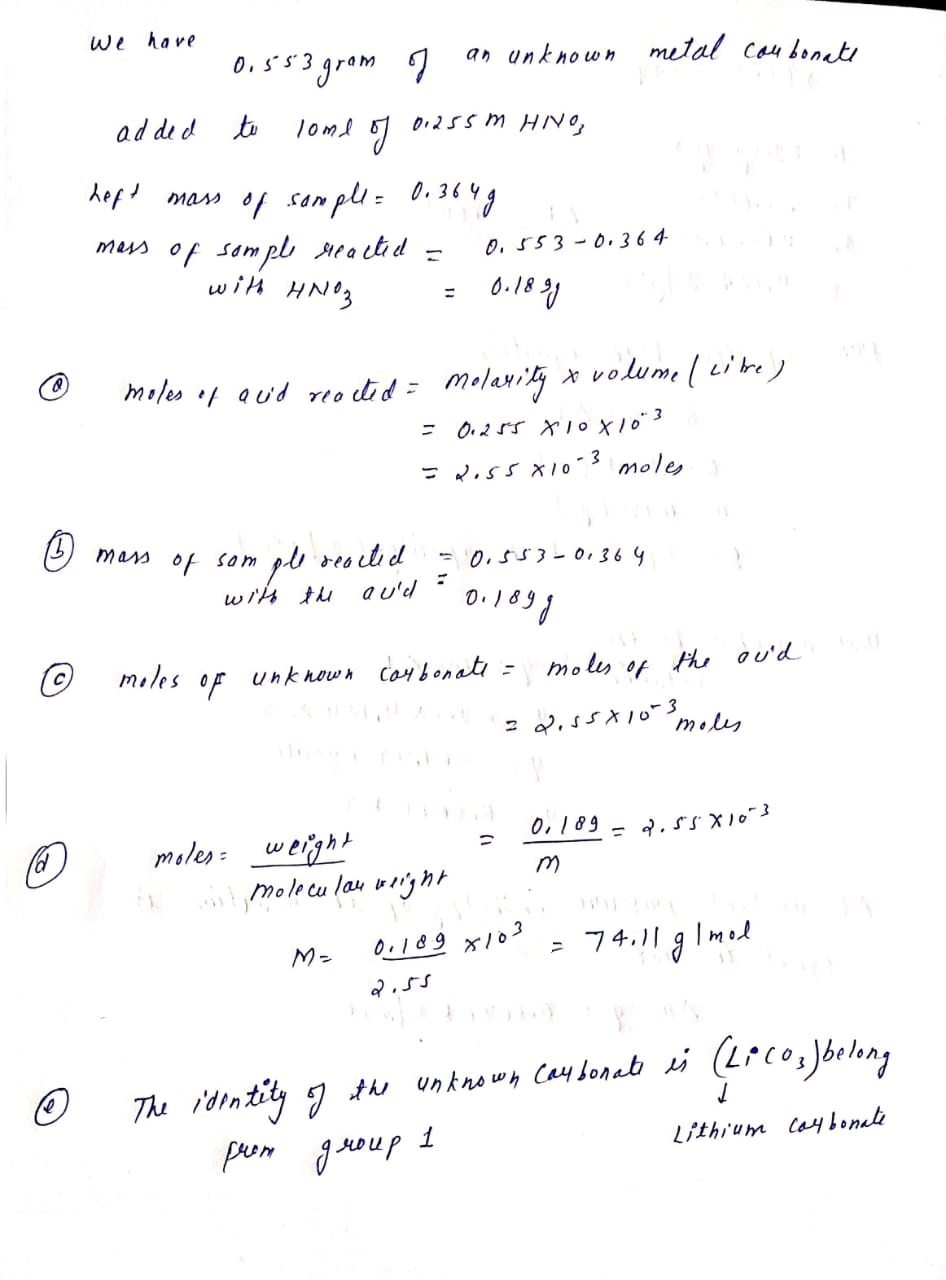#### Earn Coins

Coins can be redeemed for fabulous gifts.

Similar Homework Help Questions
• ### A carbonate salt with an unknown metal MCO3 reacts with sulfuric acid to produce MSO4, carbon...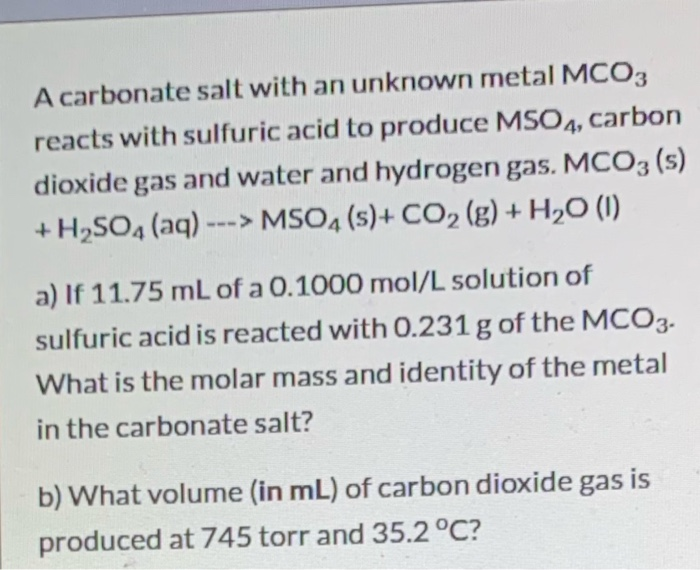A carbonate salt with an unknown metal MCO3 reacts with sulfuric acid to produce MSO4, carbon dioxide gas and water and hydrogen gas. MCO3 (s) + H2SO4 (aq) ---> MSO4(s)+ CO2(g) + H20 (1) a) If 11.75 mL of a 0.1000 mol/L solution of sulfuric acid is reacted with 0.231 g of the MCO3. What is the molar mass and identity of the metal in the carbonate salt? b) What volume (in mL) of carbon dioxide gas is produced at...

• ### A carbonate salt with an unknown metal MCO3 reacts with sulfuric acid to produce MSO4, carbon...

A carbonate salt with an unknown metal MCO3 reacts with sulfuric acid to produce MSO4, carbon dioxide gas and water and hydrogen gas. MCO3 (s) + H2SO4 (aq) ---> MSO4 (s)+ CO2 (g) + H2O (l) a) If 11.75 mL of a 0.1000 mol/L solution of sulfuric acidis reacted with 0.231 g of the MCO3. What is the molar mass and identity of the metal in the carbonate salt? b) What volume (in mL) of carbon dioxide gas is produced...

• ### A solution is made by dissolving 8.65 g sample of an unknown group 2A metal hydroxide,...

A solution is made by dissolving 8.65 g sample of an unknown group 2A metal hydroxide, M(OH)2 , in enough water to prepare 85.0 mL of solution. the unknown base reacts with hydrochloric acid according to the reaction show below. M(OH)2 (aq) +2HCl (aq) = MCl2 (aq) _ 2H2O (l) Titration of this solution required 56.9 mL of 2.50 M HCl (aq) solution to reach equivalence point. 1. What is the molar mass of the metal hydroxide? 2. What is...

• ### 5. An unknown metal carbonate was analyzed gravimetrically and yielded the following data. Calculate the values...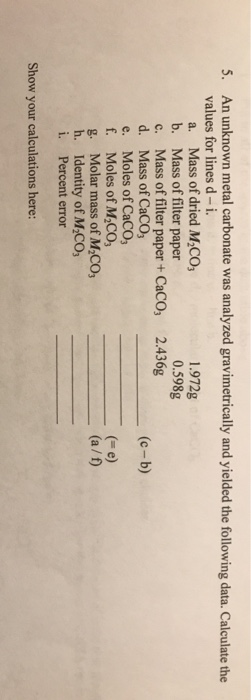5. An unknown metal carbonate was analyzed gravimetrically and yielded the following data. Calculate the values for lines d - i. a. Mass of dried M2CO3 1.972g b. Mass of filter paper 0.598g c. Mass of filter paper +CaCO3 2.436g d. Mass of CaCO3 (c-b) e. Moles of CaCO3 f. Moles of M_C03 (= e) g. Molar mass of M_C03 (a/f) h. Identity of M.CO i. Percent error Show your calculations here:

• ### A student titrates a solid sample of potassium carbonate and potassium bicarbonate that has contains 0.115...

A student titrates a solid sample of potassium carbonate and potassium bicarbonate that has contains 0.115 grams of potassium carbonate and 0.198 grams of potassium bicarbonate. a. How many moles of nitric acid are required to completely react with this sample? b. How many milliliters of 0.859 M nitric acid will be required to completely react with this sample?

• ### You analyze a sample of unknown metal using the procedure n the lab manual. Your sample...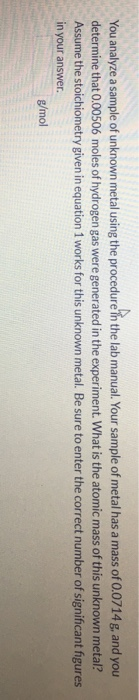You analyze a sample of unknown metal using the procedure n the lab manual. Your sample of metal has a mass of 0.0714 g, and you determine that 0.00506 moles of hydrogen gas were generated in the experiment. What is the atomic mass of this unknown metal? Assume the stoichiometry given in equation 1 works for this unknown metal. Be sure to enter the correct number of significant figures in your answer. g/mol The objective of this experiment is to...

• ### 5. As a general guideline, your experiment should look something like this: • Precisely measure the...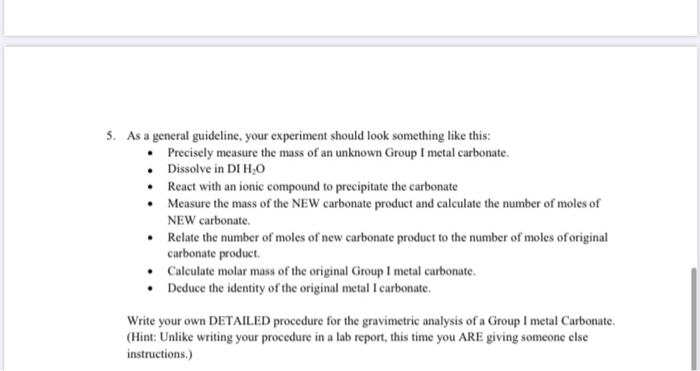5. As a general guideline, your experiment should look something like this: • Precisely measure the mass of an unknown Group I metal carbonate. • Dissolve in DI HO React with an ionic compound to precipitate the carbonate • Measure the mass of the NEW carbonate product and calculate the number of moles of NEW carbonate Relate the number of moles of new carbonate product to the number of moles of original carbonate product Calculate molar mass of the original...

• ### 3) Convert 0.200 g of sodium carbonate and 0.200 g of sodium bicarbonate to moles. Molar...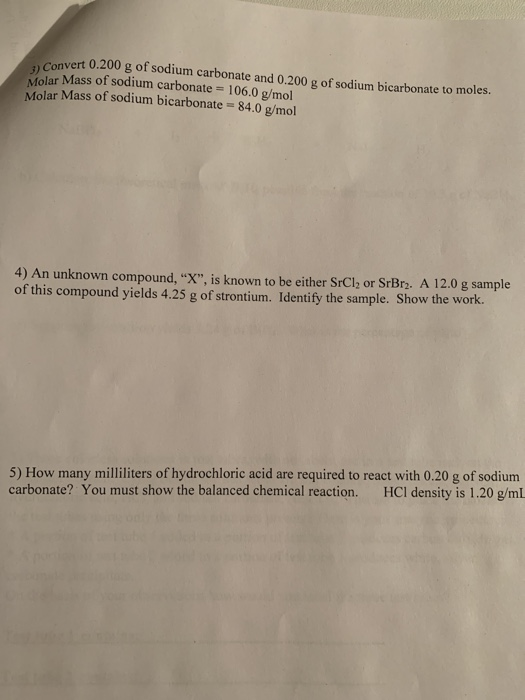3) Convert 0.200 g of sodium carbonate and 0.200 g of sodium bicarbonate to moles. Molar Mass of sodium carbonate 106.0 g/mol Molar Mass of sodium bicarbonate 84,0 g/mol 4) An unknown compound, "X", is known to be either SrCl2 or SrBr2. A 12.0 g sample of this compound yields 4.25 g of strontium. Identify the sample. Show the work. 5) How many milliliters of hydrochloric acid are required to react with 0.20 g of sodium carbonate? You must show...

• ### 2. A 0.865g sample of an unknown metal (X) reacted with hydrochloric acid according to the...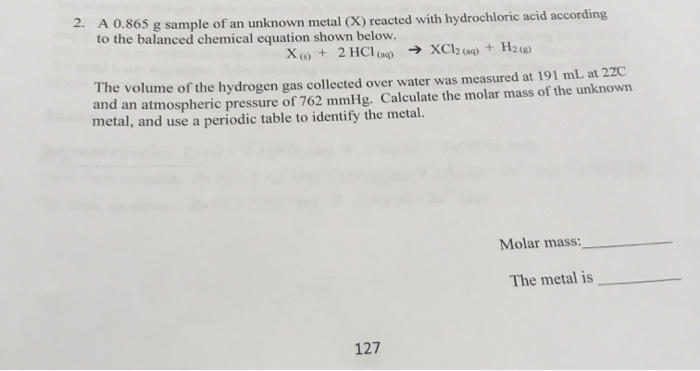2. A 0.865g sample of an unknown metal (X) reacted with hydrochloric acid according to the balanced chemical equation shown below. XC12 (aq) + H2() X+ 2 HCl(q) The volume of the hydrogen gas collected over water was measured at 191 mL at 22C and an atmospheric pressure of 762 mmHg. Calculate the molar mass of the unknown metal, and use a periodic table to identify the metal. Molar mass: The metal is 127

• ### Pre-Lab Questions 1. Write the formula and determine the molar mass for the metal carbonate of...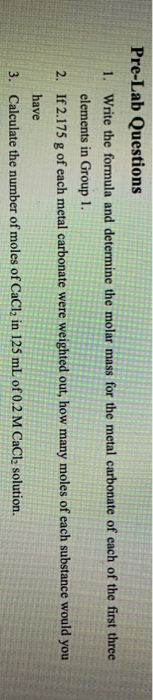Pre-Lab Questions 1. Write the formula and determine the molar mass for the metal carbonate of each of the first three elements in Group 1. 2. If 2.175 g of each metal carbonate were weighted out, how many moles of each substance would you have Calculate the number of moles of CaCl2 in 125 mL of 0.2 M CaCl solution.